# Solve thisQuestion: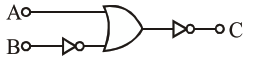The logic circuit shown above is equivalent to :

1.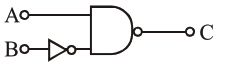2.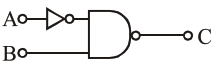3.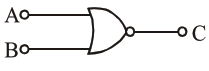4.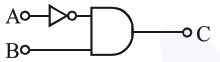Correct Option: , 4

Solution: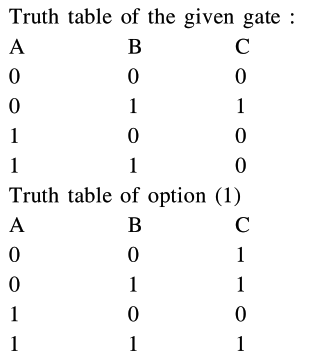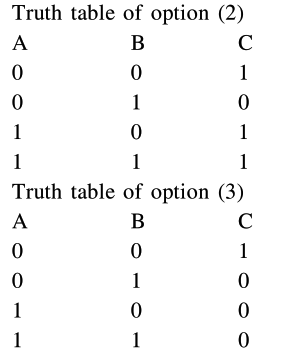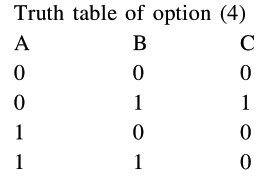Since option (1) has same truth table, hence answer is option (4) is correct.

Alternative solution :

Given Boolean expression can be written as $\overline{\mathrm{A}+\overline{\mathrm{B}}}=\mathrm{C}$

$\therefore \mathrm{C}=\overline{\mathrm{A}} \cdot \overline{\mathrm{B}}=\overline{\mathrm{A}} \cdot \mathrm{B}$